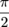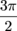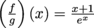Study Guide

# Continuity at a Point

## Continuity at a Point

Continuity is easiest if we begin by thinking of it at a single point. Once we have that down we can start thinking of continuity in broader terms. There's a couple conditions that have to be met for us to say a function is continuous at a point c.

The first condition is that f(c) has to actually exist. We can't have a hole in the graph at c, or an asymptote, or anything that's going to make f(c) not exist as a nice, real number.

In other words, c has to be in the domain of f.

This isn't the only condition, though. We also need.

If these two conditions are met, we say that f is continuous at x = c.

In words, the function doesn't jump around at x = c. There will be no surprises; the function will pass smoothly through x = c unscathed. The limit as we approach c will exist and be equal f(c).

### The Pencil Rule of Continuity

A continuous function is one that we can draw without lifting our pencil, pen, or Crayola crayon.

Here are some examples of continuous functions:If a function is continuous at xc we can start with our pencil a little to the left of x = c and trace the graph until our pencil is a little to the right of xc, without lifting our pencil along the way.

We will now return to those functions that are continuous at x = c. We can trace each function with a pencil, from one side of x = to the other, without lifting the pencil.

If a function isn't continuous at x = c, we say it's discontinuous at x = c.

### Sample ProblemThis function is not continuous at x = c, since the function isn't even defined at x = c. We can't compare the value of f(c) to, since neither exists!

### Sample ProblemThis function "jumps" at x = c. To draw the graph we would have to draw one line, stop at x = c and lift the pencil, then draw another line. As far as the limit definition goes,doesn't even exist (the one-sided limits disagree). Therefore f can't possibly be continuous at c.

### Sample ProblemThis function also jumps at x = c. To draw the graph we would have to draw a line, lift the pencil and draw a dot at x = c, then lift the pencil again to draw the remaining line. In this graph both f(c) andexist, but the function value disagrees with the limit.

If a function f is discontinuous at x = c, then at least one of three things need to go wrong. Either

• f(c) is undefined (therefore we can't draw it at all),

• we need to move the pencil either just before or just after we reach x = c (doesn't exist), or

• we need to move the pencil either just before or just after we reach x = c and we need to draw a separate little dot for f(c).

In other words: for a function f(x) to be continuous at x = c, three things need to happen:

• The function must be defined at x = c (that is, f(c) must exist)

• The limitmust exist (both one-sided limits must exist and agree)

• The value f(c) must agree with the limit.

• ### Continuity at a Point via Formulas

It's good to have a feel for what continuity at a point looks like in pictures. However, sometimes we're asked about the continuity of a function for which we're given a formula, instead of a picture. When this happens, remember that the following three statements must all hold for f to be continuous at c.

1. I. The function f is defined at x = c.

2. The limitexists.

3. The value f(c) agrees with the limit• ### Functions and Combinations of Functions

Many functions are continuous at every real number, x. These functions include (but are not limited to):

1. all polynomials (including lines)

2.  ex

3. sin(x) and cos(x)

It's helpful to see the continuity by graphing the functions. If we graph any of the above functions, we see a nice smooth graph that continues across the whole x-axis, with no jumps or holes. Try it; it will bring you and your TI-83 closer together.

Many other functions are continuous everywhere that they're defined, including

1. ln(x): continuous for all > 0
2. tan(x): continuous in between multiples ofand3. all rational functions that don't have common roots in the numerator and denominator: these will have vertical asymptotes at the roots of the denominator, and be continuous in between those asymptotes.

Once we know a couple of functions that are continuous at a point c, we can build other functions that are continuous at c by combining the functions we already have. To do this, we use some properties of limits.

If f and g are continuous at c, then

1. We can add or subtract:

(f + g) and (f g) are continuous at c.

2. We can multiply:

(fg) is continuous at c.

3. We can divide functions:is continuous at c as long as g(c) ≠ 0.

4. We can compose:

The composition (f ο g) is continuous at c.

All that's required here is that we have two functions continuous at c. It doesn't matter which is f and which is g. By switching f and g in our minds, we also find that (g f) is continuous at c, g ο f is continuous at c, etc.

### Sample Problem

Let f(x) = x + 1 and g(x) = ex. These functions are both continuous at every real number x. The following functions are also continuous at every real number x:

1. We can add or subtract:

(f + g)(x) = x + 1 + ex (which is the same as (g + f)(x))

(fg)(x) = (x + 1) – ex

(g f)(x) = ex – (x + 1) = ex x – 1

2. We can multiply:

(fg)(x) = (x + 1)(ex) = xex + ex (which is the same as (gf)(x))

3. We can divide:(This is continuous at every real number since ex is never 0.)

4. We can compose:

(f ο g)(x) = (ex) + 1

(g ο f)(x) = ex + 1

Also, the functionis continuous at every real number except x = -1.